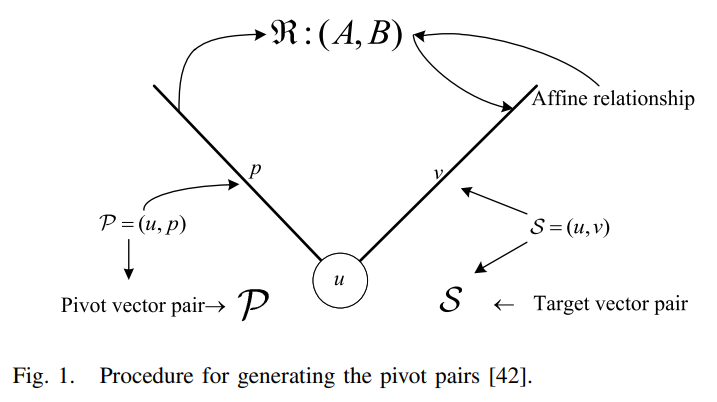Journal

# Dominant Data Set Selection Algorithms for Electricity Consumption Time-Series Data Analysis Based on Affine Transformation

By
Wu Y.
Liu Y.
Ahmed S.H.
Peng J.
El-Latif A.A.A.

In the explosive growth of time-series data (TSD), the scale of TSD suggests that the scale and capability of many Internet of Things (IoT)-based applications has already been exceeded. Moreover, redundancy persists in TSD due to the correlation between information acquired via different sources. In this article, we propose a cohort of dominant data set selection algorithms for electricity consumption TSD with a focus on discriminating the dominant data set that is a small data set but capable of representing the kernel information carried by TSD with an arbitrarily small error rate less than $\varepsilon$. Furthermore, we prove that the selection problem of the minimum dominant data set is an NP-complete problem. The affine transformation model is introduced to define the linear correlation relationship between TSD objects. Our proposed framework consists of the scanning selection algorithm with $O({n^{3}})$ time complexity and the greedy selection algorithm with $O({n^{4}})$ time complexity, which are, respectively, proposed to select the dominant data set based on the linear correlation distance between TSD objects. The proposed algorithms are evaluated on the real electricity consumption data of Harbin city in China. The experimental results show that the proposed algorithms not only reduce the size of the extracted kernel data set but also ensure the TSD integrity in terms of accuracy and efficiency. © 2014 IEEE.